# Fan

The fan has a speed of 210 RPM. Calculate for time of one fan period.

Result

T =  0.3 s

#### Solution:Leave us a comment of example and its solution (i.e. if it is still somewhat unclear...):Be the first to comment!## Next similar examples:

1. A trip to the peak Dry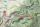Calculate what percentage is rising trip from Strečno to peak Suchý (Dry peak) longer than the descent back. Team trio of tourists (Palo, Peter and Andrew) rise took 4:21 and descent 3:08.
2. Pump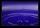From the pump in the yard dripping water. Every drop is 1 ml. How many drops will be necessary before filling a bucket with a volume of 10 l? Calculate how long it will fulfill the bucket when drops fall each second.
3. AreaCalculate: ?
4. WorkersThree factory operators produced 480 units in 50 minutes. How many hours worked? I'm trying to prove or disapprove the idea that the company tell me that it's 2.5 hours. So what is right? Thank you, Petra
5. HoursThe lesson lasts 45 minutes. For the week, students have 18 lesson hours. How many are the actual hours?
6. Hr to minSue biked to school in 5/12 of an hour. How many minutes did it take her to ride to school?
7. Clock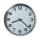How many hours are 15 days?
8. BreederSeven hens breeder has supplied 350 eggs. How long can deliver, if each of its chickens can withstand at least 5 eggs for week?
9. The shopThe shop has 3 hectoliters of water. How many liter bottles is it?
10. The resultHow many times I decrease the number 1632 to get the result 24?
11. Pizza 5You have 2/4 of a pizza and you want to share it equally between 2 people how much pizza does each person get?
12. Crates 2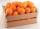One crate will hold 50 oranges. If Bob needs to ship 932 oranges, how many crates will he need?
13. Sweets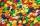Mom bought box of sweets for their children.Whole package of 100 sweets divided among 4 their children so that each child receives the most and for she remains the least sweets. How many sweets left for mom.
14. Math classificationIn 3A class are 27 students. One-third got a B in math and the rest got A. How many students received a B in math?
15. Fractions 4How many 2/3s are in 6?
16. Date game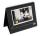Let now is Wednesday. What day is after 248 days? Write the result as a number: 1=Monday, 2=Tuesday, 3=Wednesday, 4=Thursday, 5=Friday, 6=Saturday, 7=Sunday?
17. Product of two fractionsProduct of two fractions is 9 3/5 . If one of the fraction is 9 3/7. Find the other fraction.